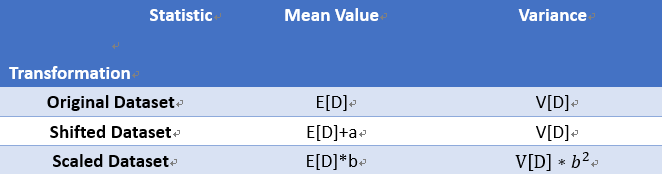#2019 iT 邦幫忙鐵人賽DAY 9
0

## Day 9-機器學習與數學天天玩-PCA-Statistical Introduction: Linear Transformation Final Summary

The brief structure leading to the milestone of PCA is as below:

1. Statistical Introduction
2. Transformation of Vectors in Spaces
3. Orthogonal Projection

Friday night, let's do the final part for the linear transformation in the section of statistical introduction. In the past two days, we talked about the linear transformation of mean value (How mean value varies when the original dataset is shifted or scaled.) Now, think about what impact the linear transformation brings on variance.The shifted dataset has no impact on the original variance as the difference among each data point remains the same. However, the scaled dataset has an enlarged impact on the original variance because of the squared computation.

Not being easy to find extra time to write everything in detail, let's try to move forward and conquer the busy period! Fighting!